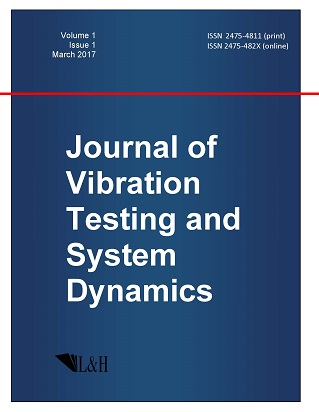ISSN: 2475-4811 (print)
ISSN: 2475-482X (online)
Journal of Vibration Testing and System Dynamics

C. Steve Suh (editor), Pawel Olejnik (editor),

Xianguo Tuo (editor)

Pawel Olejnik (editor)

Lodz University of Technology, Poland

Email: pawel.olejnik@p.lodz.pl

C. Steve Suh (editor)

Texas A&M University, USA

Email: ssuh@tamu.edu

Xiangguo Tuo (editor)

Sichuan University of Science and Engineering, China

Email: tuoxianguo@suse.edu.cn

Existence and Uniqueness of Solutions for the Neutral Fractional Integro-Differential Equations with Fractional Integral Boundary Conditions

Journal of Vibration Testing and System Dynamics 6(1) (2022) 1--11 | DOI:10.5890/JVTSD.2022.03.001

Ahmed A. Hamoud$^{1}$ , Abdulrahman A. Sharif$^{2}$, Kirtiwant P. Ghadle$^{3}$

$^{1}$ Department of Mathematics, Taiz University, Taiz, Yemen

$^{2}$ Department of Mathematics, Hodeidah University, Al-Hodeidah, Yemen

$^{3}$ Department of Mathematics, Dr. Babasaheb Ambedkar Marathwada University, Aurangabad, India

Abstract

In this paper, we establish sufficient conditions for the existence and uniqueness of solutions for a class of boundary value problems with integral condition for neutral fractional integro-differential equations. The results are established by the application of the contraction mapping principle, Leray-Schauder alternative theorem and Krasnoselskii fixed point theorem. An example is provided to illustrate the main results.

Acknowledgments

The authors would like to thank the referees and the editor of this journal for their valuable suggestions and comments that improved this paper.

References

1.   Bani Issa, M., Hamoud, A., and Ghadle, K. (2021), Numerical solutions of fuzzy integro-differential equations of the second kind, Journal of Mathematics and Computer Science, 23(1), 67-74.
2.   Hamoud, A. (2020), Existence and uniqueness of solutions for fractional neutral Volterra-Fredholm integro differential equations, Advances in the Theory of Nonlinear Analysis and its Application, 4(4), 321-331.
3.   Dawood, L., Hamoud, A., and Mohammed, N. (2020), Laplace discrete decomposition method for solving nonlinear Volterra-Fredholm integro-differential equations, J. Math. Comput. SCI., 21(2), 158-163.
4.   Hamoud, A. and Ghadle, K. (2019), Some new existence, uniqueness and convergence results for fractional Volterra-Fredholm integro-differential equations, J. Appl. Comput. Mech., 5(1), 58-69.
5.   Kilbas, A., Srivastava, H. and Trujillo, J. (2006), Theory and Applications of Fractional Differential Equations, North-Holland Math. Stud., 204, Elsevier, Amsterdam.
6.   Ahmad, B. and Sivasundaram, S. (2008), Some existence results for fractional integro-differential equations with nonlinear conditions, Communications Appl. Anal., 12, 107-112.
7.   Matar, M. (2010), Controllability of fractional semilinear mixed Volterra-Fredholm integro differential equations with nonlocal conditions, Int. J. Math. Anal., 4(23), 1105-1116.
8.   Miller, K. and Ross, B. (1993), An Introduction to the Fractional Calculus and Differential Equations, John Wiley, New York.
9.   Ibrahim, R. and Momani, S. (2007), On the existence and uniqueness of solutions of a class of fractional differential equations, Journal of Mathematical Analysis and Applications, 334(1), 1-10.
10.   Wu, J. and Liu, Y. (2010), Existence and uniqueness results for fractional integro-differential equations with nonlocal conditions, 2nd IEEE International Conference on Information and Financial Engineering, 91-94.
11.   Karthikeyan, K. and Trujillo, J. (2012), Existence and uniqueness results for fractional integro-differential equations with boundary value conditions, Commun. Nonlinear Sci. Numer. Simulat., 17, 4037-4043.
12.   Bahuguna, D. and Dabas, J. (2008), Existence and uniqueness of a solution to a partial integro-differential equation by the method of Lines, Electronic Journal of Qualitative Theory of Differential Equations, 4, 1-12.
13.   Ahmad, B., Ntouyas, S.K., Alsaedi, A., and Alnahdi, M. (2018), Existence theory for fractional-order neutral boundary value problems, Frac. Differ. Calc., 8, 111-126.
14.   Ahmad, B., Ntouyas, S.K., and Alsaedi, A. (2017), Caputo-type fractional boundary value problems for differential equations and inclusions with multiple fractional derivatives, J. Nonlinear Funct. Anal., 2017, Art. ID 52.
15.   Ntouyas, S.K. (2013), Existence results for nonlocal boundary value problems for fractional differential equations and inclusions with fractional integral boundary conditions, Discuss. Math. Differ. Incl. Control Optim., 33, 17-39.
16.   Balachandran, K. and Trujillo, J. (2010), The nonlocal Cauchy problem for nonlinear fractional integro-differential equations in Banach spaces, Nonlinear Anal. Theory Meth. Applic., 72, 4587-4593.
17.   Balachandran, K. and Uchiyama, K. (2000), Existence of solutions of nonlinear integro-differential equations of Sobolev type with nonlocal condition in Banach spaces, Proceedings of the Indian Academy of Science, 110, 225-232.
18.   Hamoud, A., Mohammed, N., and Ghadle, K. (2020), Existence and uniqueness results for Volterra-Fredholm integro-differential equations, Advances in the Theory of Nonlinear Analysis and its Application, 4(4), 361-372.
19.   Hamoud, A., Hussain, K., and Ghadle, K. (2019), The reliable modified Laplace Adomian decomposition method to solve fractional Volterra-Fredholm integro-differential equations, Dynamics of Continuous, Discrete and Impulsive Systems, Series B: Applications and Algorithms, 26, 171-184.
20.   Hamoud, A. and Ghadle, K. (2018), The approximate solutions of fractional Volterra-Fredholm integro-differential equations by using analytical techniques, Probl. Anal. Issues Anal., 7(25)(1), 41-58.
21.   Hamoud, A. and Ghadle, K. (2018), Modified Laplace decomposition method for fractional Volterra-Fredholm integro-differential equations, J. Math. Model., 6(1), 91-104.
22.   Hamoud, A. and Ghadle, K. (2018), Usage of the homotopy analysis method for solving fractional Volterra-Fredholm integro-differential equation of the second kind, Tamkang J. Math., 49(4), 301-315.
23.   Hamoud, A. and Ghadle, K. (2018), Existence and uniqueness of solutions for fractional mixed Volterra-Fredholm integro-differential equations, Indian J. Math., 60(3), 375-395.
24.   Lakshmikantham, V. and Rao, M. (1995), Theory of Integro-Differential Equations, Gordon \& Breach, London.
25.   Samko, S., Kilbas, A., and Marichev, O. (1993), Fractional Integrals and Derivatives, Theory and Applications, Gordon and Breach, Yverdon.
26.   Wu, J. and Liu, Y. (2009), Existence and uniqueness of solutions for the fractional integro-differential equations in Banach spaces, Electronic Journal of Differential Equations, 2009, 1-8.